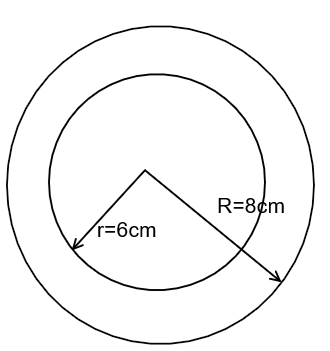Courses
Courses for Kids
Free study material
Free LIVE classes
More# Find the area of the annulus whose inner and outer radii are 6cm and 8cm.

Last updated date: 18th Mar 2023
Total views: 204.9k
Views today: 1.84kVerified
204.9k+ views
Hint: The area of the annulus, we will use: $\pi \left( {{R^2} - {r^2}} \right)$where the outer radii is the R and the inner radii is the ‘r’ to find the required area. The value of the inner and the outer radii we will substitute and then simplify it to get the required answer.

Complete step-by-step solution:
We have to find the area of the annulus whose inner and outer radii are 6cm and 8cm. The area of the annulus, we know is $\pi \left( {{R^2} - {r^2}} \right)$, where the r is the outer radii and ‘r’ is the inner radii.In the above formula, we will substitute the values of inner radii and outer radii.
$A= \pi \left( {\left(8^2\right)}-{\left(6^2\right)} \right)$
By using the formula we will simplify the above expression,
${a^2} - {b^2} = \left( {a + b} \right)\left( {a - b} \right)$
$\Rightarrow A=\pi \left( {8 + 6} \right)\left( {8 - 6} \right) = \pi \left( {14} \right)\left( 2 \right)$
$\Rightarrow A= \pi \left( {14} \right)\left( 2 \right)$
On substituting the value of the $\pi = \dfrac{{22}}{7}$ in the above expression, we will get,
$\Rightarrow A= \dfrac{{22}}{7}\left( {14} \right)\left( 2 \right)$
$\Rightarrow A= 22\left( 4 \right)$
$\Rightarrow A= 88c{m^2}$
Hence, the area of the annulus is $88c{m^2}$.

Note: By finding the area of the outer circle and the inner circle, we can also do this question, and then we can subtract the area of the inner circle from the area of the outer circle. Between the two circles, the annulus is the part. Also the area of any shape is measured in square units.